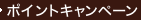•### A First Course in Differential Equations : With Modeling Applications （9TH）

• ただいまウェブストアではご注文を受け付けておりません。 ⇒古書を探す
• 製本 Hardcover:ハードカバー版／ページ数 362 p.
• 言語 ENG,ENG
• 商品コード 9780495108245
• DDC分類 515.35

### Table of Contents

`Preface                                            ix    Introduction to Differential Equations         1  (33)      Definitions and Terminology                  2  (11)      Initial-Value Problems                       13 (6)      Differential Equations as Mathematical       19 (15)      Models        in Review                                  32 (2)    First-Order Differential Equations             34 (48)      Solution Curves Without a Solution           35 (9)        Direction Fields                           35 (2)        Autonomous First-Order DEs                 37 (7)      Separable Variables                          44 (9)      Linear Equations                             53 (9)      Exact Equations                              62 (8)      Solutions by Substitutions                   70 (5)      A Numerical Method                           75 (7)        in Review                                  80 (2)    Modeling with First-Order Differential         82 (35)    Equations      Linear Models                                83 (11)      Nonlinear Models                             94 (11)      Modeling with Systems of First-Order DEs     105(12)        in Review                                  113(4)    Higher-Order Differential Equations            117(64)      Preliminary Theory---Linear Equations        118(12)        Initial-Value and Boundary-Value           118(2)        Problems        Homogeneous Equations                      120(5)        Nonhomogeneous Equations                   125(5)      Reduction of Order                           130(3)      Homogeneous Linear Equations with            133(7)      Constant Coefficients      Undetermined Coefficients---Superposition    140(10)      Approach      Undetermined Coefficients---Annihilator      150(7)      Approach      Variation of Parameters                      157(5)      Cauchy-Euler Equation                        162(7)      Solving Systems of Linear DEs by             169(5)      Elimination      Nonlinear Differential Equations             174(7)        in Review                                  178(3)    Modeling With Higher-Order Differential        181(38)    Equations      Linear Models: Initial-Value Problems        182(17)        Spring/Mass Systems: Free Undamped         182(4)        Motion        Spring/Mass Systems: Free Damped Motion    186(3)        Spring/Mass Systems: Driven Motion         189(3)        Series Circuit Analogue                    192(7)      Linear Models: Boundary-Value Problems       199(8)      Nonlinear Models                             207(12)        in Review                                  216(3)    Series Solutions of Linear Equations           219(36)      Solutions About Ordinary Points              220(11)        Review of Power Series                     220(3)        Power Series Solutions                     223(8)      Solutions About Singular Points              231(10)      Special Functions                            241(14)        Bessel's Equation                          241(7)        Legendre's Equation                        248(5)        in Review                                  253(2)    The Laplace Transform                          255(48)      Definition of the Laplace Transform          256(6)      Inverse Transforms and Transforms of         262(8)      Derivatives        Inverse Transforms                         262(3)        Transforms of Derivatives                  265(5)      Operational Properties I                     270(12)        Translation on the s-Axis                  271(3)        Translation on the t-Axis                  274(8)      Operational Properties II                    282(10)        Derivatives of a Transform                 282(1)        Transforms of Integrals                    283(4)        Transform of a Periodic Function           287(5)      The Dirac Delta Function                     292(3)      Systems of Linear Differential Equations     295(8)        in Review                                  300(3)    Systems of Linear First-Order Differential     303(36)    Equations      Preliminary Theory---Linear Systems          304(7)      Homogeneous Linear Systems                   311(15)        Distinct Real Eigenvalues                  312(3)        Repeated Eigenvalues                       315(5)        Complex Eigenvalues                        320(6)      Nonhomogeneous Linear Systems                326(8)        Undetermined Coefficients                  326(3)        Variation of Parameters                    329(5)      Matrix Exponential                           334(5)        in Review                                  337(2)    Numerical Solutions of Ordinary                339(1)    Differential Equations      Euler Methods and Error Analysis             340(5)      Runge-Kutta Methods                          345(5)      Multistep Methods                            350(3)      Higher-Order Equations and Systems           353(5)      Second-Order Boundary-Value Problems         358(4)        in Review                                  362  Appendices      Gamma Function                               1  (2)      Matrices                                     3  (18)      Laplace Transforms                           21Answers for Selected Odd-Numbered Problems         1Index`